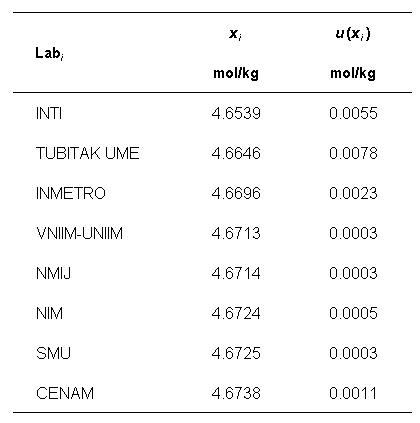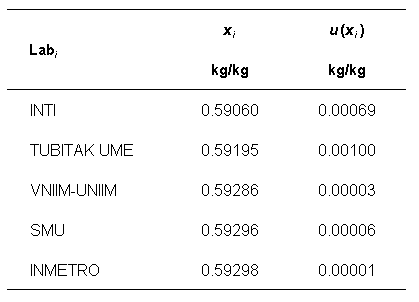Comparison
Comparison close
Results

CCQM-K152

Assay of Potassium Iodate

MEASURAND          Amount content of oxidants expressed as potassium iodate

NOMINAL VALUE        4.65 mol/kg

Degrees of equivalence represented by Di = (xI - xR) and its expanded uncertainty Ui at a 95 % level of confidence, both expressed in mol/kg.

Amount content
Di (mol/kg)
Participating institutes

CCQM-K152

Assay of Potassium Iodate

MEASURAND          Mass fraction of iodine

NOMINAL VALUE        0.59 kg/kg

Degrees of equivalence represented by Di = (xI - xR) and its expanded uncertainty Ui at a 95 % level of confidence, both expressed in kg/kg.

Mass fraction
Di (kg/kg)
Participating institutes
Comparison
Comparison close
Results

CCQM-K152

Assay of Potassium Iodate

MEASURAND          Amount content of oxidants expressed as potassium iodate

NOMINAL VALUE        4.65 mol/kg

Degrees of equivalence represented by Di = (xI - xR) and its expanded uncertainty Ui at a 95 % level of confidence, both expressed in mol/kg.

LABi Di Uneg,i Upos,i
mol/kg
mol/kg
mol/kg
INTI -0.0180 0.0110
TUBITAK UME -0.0074 0.0160
INMETRO -0.0023 0.0046
VNIIM-UNIIM -0.0006 0.0007
NMIJ -0.0005 0.0007
NIM 0.0005 0.0010
SMU 0.0005 0.0006
CENAM 0.0019 0.0021
Participating institutes
CCQM-K152

CCQM-K152

Assay of Potassium Iodate

MEASURAND          Mass fraction of iodine

NOMINAL VALUE        0.59 kg/kg

Degrees of equivalence represented by Di = (xI - xR) and its expanded uncertainty Ui at a 95 % level of confidence, both expressed in kg/kg.

LABi Di Uneg,i Upos,i
kg/kg
kg/kg
kg/kg
INTI -0.00231 0.0014
TUBITAK UME -0.00096 0.0021
VNIIM-UNIIM -0.00005 0.0001
SMU 0.00005 0.0002
INMETRO 0.00007 0.0001
Participating institutes
CCQM-K152
Comparison
Comparison close
CC comparison
 Metrology area, Sub-field Chemistry and Biology, Inorganics Description Assay of Potassium Iodate Time of measurements 2019 Status Approved for equivalence
 Final Reports of the comparisons Measurand Mass fraction of iodine, kg/kg Amount content of oxidants expressed as potassium iodate, mol/kg From 0.592 kg/kg to 0.597 kg/kg From 4.65 mol/kg to 4.68 mol/kg Transfer device High purity potassium iodate sample
 Comparison type Key Comparison Consultative Committee CCQM (Consultative Committee for Amount of Substance) Conducted by CCQM (Consultative Committee for Amount of Substance) Pilot institute VNIIM D.I. Mendeleyev Institute for Metrology, Rosstandart Russian Federation Contact person Alena Sobina +73432280012
First Name Last Name
wwww@ww.www +356719836 Institute 1 Institute 1 Khmelnitskiy
Pilot laboratory
VNIIM

D.I. Mendeleyev Institute for Metrology, Rosstandart, Russian Federation, COOMET

 CENAM Centro Nacional de Metrologia, Mexico, SIM
 INMETRO Instituto Nacional de Metrologia, Qualidade e Tecnologia, Brazil, SIM
 INTI Instituto Nacional de Tecnologia Industrial, Argentina, SIM
 NIM National Institute of Metrology, China, APMP
 NMIJ AIST National Metrology Institute of Japan, Japan, APMP
 SMU Slovensky Metrologicky Ustav, Slovakia, EURAMET
 UME TÜBITAK Ulusal Metroloji Enstitüsü, Türkiye, EURAMET
Comparison
Comparison close
Results

CCQM-K152

Assay of Potassium Iodate

MEASURAND          Amount content of oxidants expressed as potassium iodate

NOMINAL VALUE        4.65 mol/kg

The key comparison reference value xR and its associated standard uncertainty uR are computed from a weighted mean of the reported results, INTI excluded.

xR = 4.67194 mol/kg     uR = 0.00025 mol/kg

The degree of equivalence of laboratory i relative to the key comparison reference value xR is given by a pair of terms, both expressed in mol/kg:

Di = (xi - xR) and its expanded uncertainty Ui at a 95 % level of confidence where Ui = 2 ui.

CCQM-K152

Assay of Potassium Iodate

MEASURAND          Mass fraction of iodine

NOMINAL VALUE        0.59 kg/kg

The key comparison reference value xR and its associated standard uncertainty uR are computed from a weighted mean of the reported results, INTI excluded.

xR = 0.592 91 kg/kg     uR = 0.000 05 kg/kg

The degree of equivalence of laboratory i relative to the key comparison reference value xR is given by a pair of terms, both expressed in kg/kg:

Di = (xi - xR) and its expanded uncertainty Ui at a 95 % level of confidence where Ui = 2 ui.

Comparison
Comparison close
Results

CCQM-K152

Assay of Potassium Iodate

MEASURAND          Amount content of oxidants expressed as potassium iodate

NOMINAL VALUE        4.65 mol/kg

xi   measured amount of content reported by laboratory iLabi

ui   combined standard uncertainty of xiCCQM-K152

Assay of Potassium Iodate

MEASURAND          Mass fraction of iodine

NOMINAL VALUE        0.59 kg/kg

xi   measured mass fraction reported by laboratory iLabi

ui   combined standard uncertainty of xi